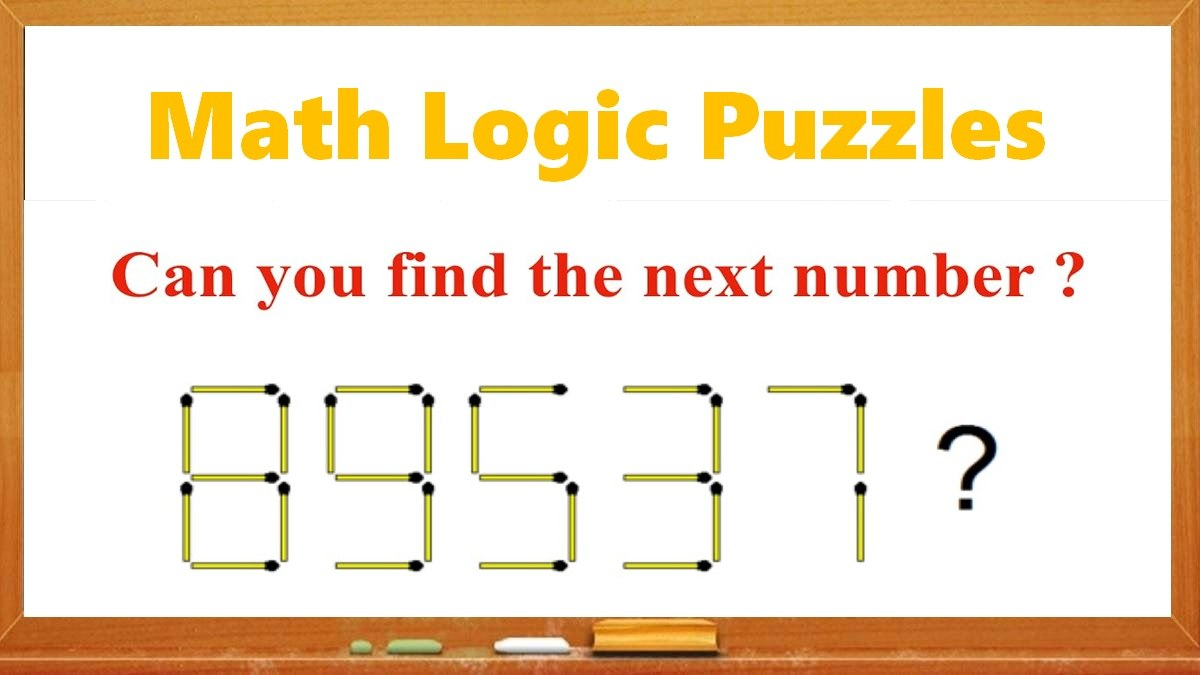# Math Riddles: Solve These Hard Logic Puzzles in 20 Seconds Each

Math Riddles: Find out how brilliant you are. Only 1% of people with a high IQ can find the missing numbers in these tricky logic math puzzles in 20 seconds each.Math Riddles: Solve These Hard Logic Puzzles in 20 Seconds Each

Math Riddles are so challenging, but that makes them worthwhile to solve. Math riddles are logical problems that require strong analytical abilities, high IQ, knowledge of math concepts, and good calculation skills. Math riddles have also been known to make Mathematics enjoyable for kids, students, and even adults to solve. Riddles, puzzles, and brain teasers can develop strategic thinking, logical reasoning, and problem-solving skills.

Today, we bring you another set of math puzzles that is all over social media. These Tricky Logic Math Puzzles have attracted millions of answers but very few got it right.

Can you solve these hard math puzzles? You have 20 seconds!

## Tell us in comments: Did you solve these math puzzles correctly in 20 seconds each?

Check out more math puzzles!

Also Read: Math Riddles: Solve These Hard Logic Puzzles in 20 Seconds Each

Also Read: Math Riddles: Solve these Tricky Logic Puzzles in 20 seconds each

Also Read: Math Riddles: Find Missing Numbers in these Tricky Logic Math Puzzles

Also Read: Math Riddles with Answers: Can You Solve These Tricky Math Puzzles?

Also Read: Math Riddles: Can You Solve This Viral ‘Apple, Banana, Orange’ Picture Puzzle in 20 Seconds?

Get the latest General Knowledge and Current Affairs from all over India and world for all competitive exams.
खेलें हर किस्म के रोमांच से भरपूर गेम्स सिर्फ़ जागरण प्ले पर